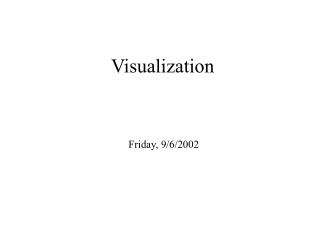DownloadDownload PresentationVisualization

# Visualization

Download Presentation## Visualization

- - - - - - - - - - - - - - - - - - - - - - - - - - - E N D - - - - - - - - - - - - - - - - - - - - - - - - - - -
##### Presentation Transcript

1. Visualization Friday, 9/6/2002

2. Hedgehog Method

3. MATLAB functions plot Example x = 0:pi/100:2*pi; y = sin(x); plot(x,y)

4. Bean-Bag Method

5. MATLAB functions meshgrid mesh Examples: [x,y]=meshgrid(-10:10,-10:10); [r,theta]=meshgrid(0:5:10,0:pi/20:pi); z=sqrt(x.^2+y.^2); mesh(x,y,z)

6. Sphere Scanning theta = linspace(0,2*pi,ntheta); phi = linspace(0,pi,nphi); [x,y] = meshgrid( theta, phi ); for i=1:nphi for j=1:ntheta n = [ sin(phi(i))*cos(theta(j)), ... sin(phi(i))*sin(theta(j)), ... cos(phi(i)) ]'; x(i,j) = … … ; y(i,j) = … … ; z(i,j) = … … ; end end mesh(x,y,z)

7. Hole Problem

8. Stress Field Tangential stress Radial stress

9. Cymbals

10. Stress Field Around Crack Tip Thin plate

11. Von Mises’ Yield Criterion Perfect plasticity

12. Crack Tip Stress Field

13. Mountain Stream A mountain stream comes trickling in the sunlight down the hill, And gathers volume until it has strength to run the mill; It happily continues then, upon its useful way, Turns other mills still further down, until it joins the bay.

14. Dislocation Emissions